Function Repository Resource:

# NonNegativeExcessDistribution

Compute the distribution of the amount in excess of a specific value drawn from an underlying distribution

Contributed by: Seth J. Chandler
 ResourceFunction["NonNegativeExcessDistribution"][value,dist] creates a distribution representing the non-negative amount in excess of value drawn from the distribution dist.

## Details

The term "non-negative excess" means the amount not less than zero by which a certain value exceeds another value. Thus, the non-negative excess of 9 over 5 is 4, and the non-negative excess of 3 over 4 is 0.
ResourceFunction["NonNegativeExcessDistribution"] can be useful in modeling the distribution of financial responsibility of higher tiers of insurance (such as excess insurance) or of policyholders for amounts exceeding the limits of insurance.

## Examples

### Basic Examples (3)

Compute the distribution of the amount in excess of 2/3 for a uniform distribution on the interval from 0 to 1:

 In:=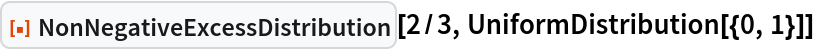Out=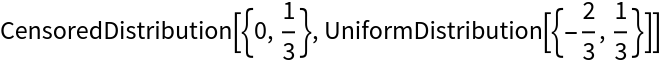Compute the mean of the excess:

 In:=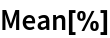Out=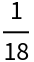Compute the distribution of the amount in excess of a point for a uniform distribution in four dimensions:

 In:=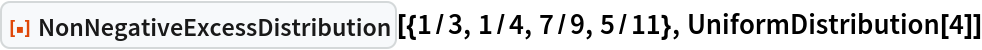Out=Compute the mean of the excess:

 In:=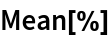Out=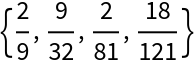Compute the distribution of the amount in excess of 10 for a log-normal distribution with a mean of 9 and a median of 3:

 In:=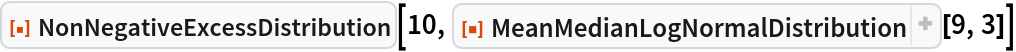Out=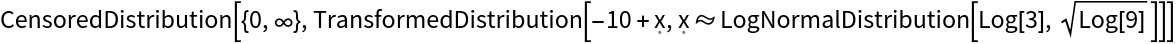Compute the mean and standard deviation:

 In:=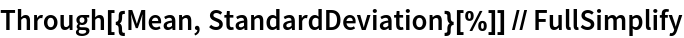Out=### Scope (2)

The function works with discrete distributions:

 In:=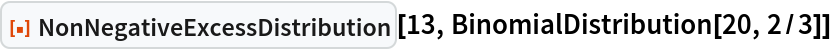Out=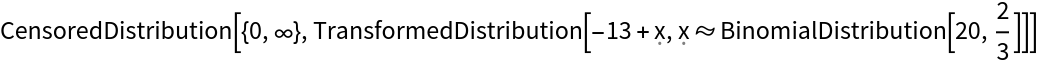In:=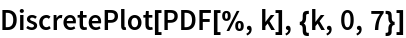Out=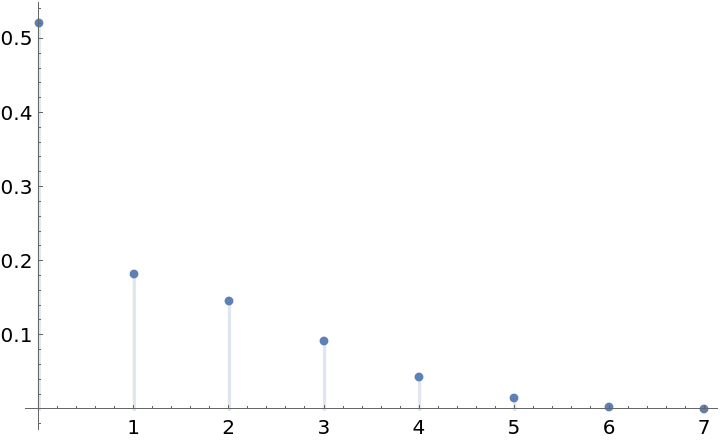One can compute a symbolic non-negative excess distribution from an underlying symbolic univariate distribution:

 In:=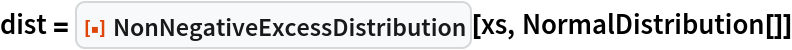Out=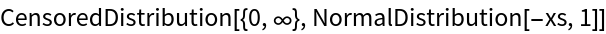Compute various statistics of the resulting distribution:

 In:=Out=Calculate for a numeric value:

 In:=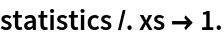Out=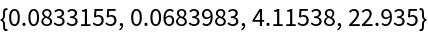### Properties and Relations (2)

One can nest non-negative excess distributions. Compute the mean amount owed by an insured who has a primary policy that covers up to 2/3 and a secondary policy that will pay any amount remaining (but not exceeding 1/10) after the primary has paid its 2/3:

 In:=Out=In:=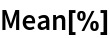Out=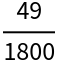The result is no different than if there was a primary policy with a limit of 2/3 and 1/10:

 In:=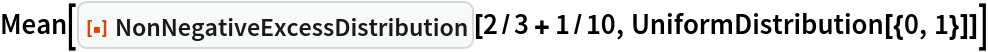Out=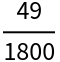### Applications (2)

An excess insurer agrees to pay for damages in excess of \$250,000 suffered by an insured where the distribution of losses is logarithmic, the mean loss is \$10,000, and the median loss is \$2,800. What premium would cause the excess insurer to break even:

 In:=Out=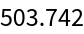A reinsurer agrees to pay 80% of the excess insurer's loss that is in turn over \$100,000. What premium would cause the reinsurer to break even:

 In:=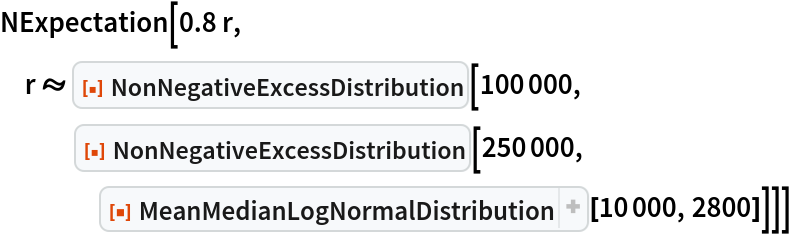Out=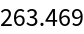### Possible Issues (2)

A mismatch between the dimension of value and the dimension of the distribution dist will gives an invalid distribution:

 In:=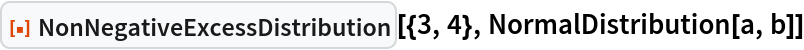Out=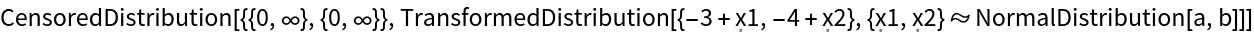When one attempts to work with the resulting distribution errors can occur:

 In:=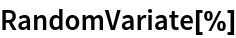Out=In:=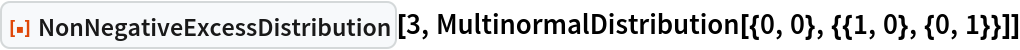Out=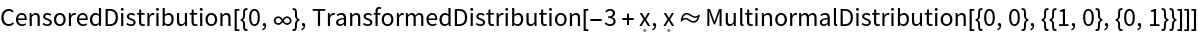In:=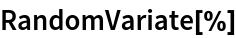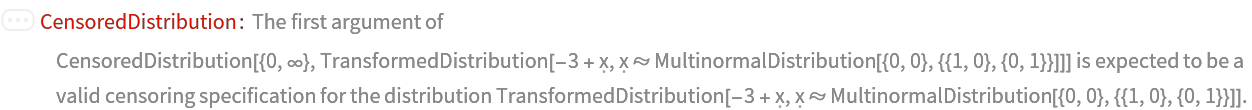Out=### Neat Examples (6)

Consider a distribution of judgments in a lawsuit that may be modeled as a log-normal distribution:

 In:=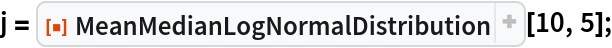Imagine an insurance policy with a limit of 20 and consider the expected positions of the insurer and insured:

 In:=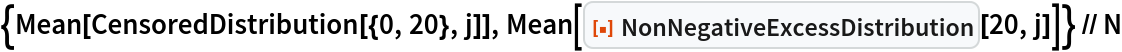Out=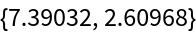Now, consider a second distribution with the same mean but a higher median:

 In:=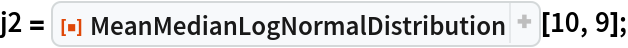Examine the positions of the insurer and the insured:

 In:=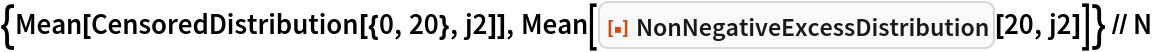Out=Note the conflict in perspectives. From the perspective of the insurer, the second distribution leaves them in a worse expected position. From the perspective of the insured, the second distribution leaves them in a better position. Plot the difference in case values as the median goes from 1 to 9.9:

 In:=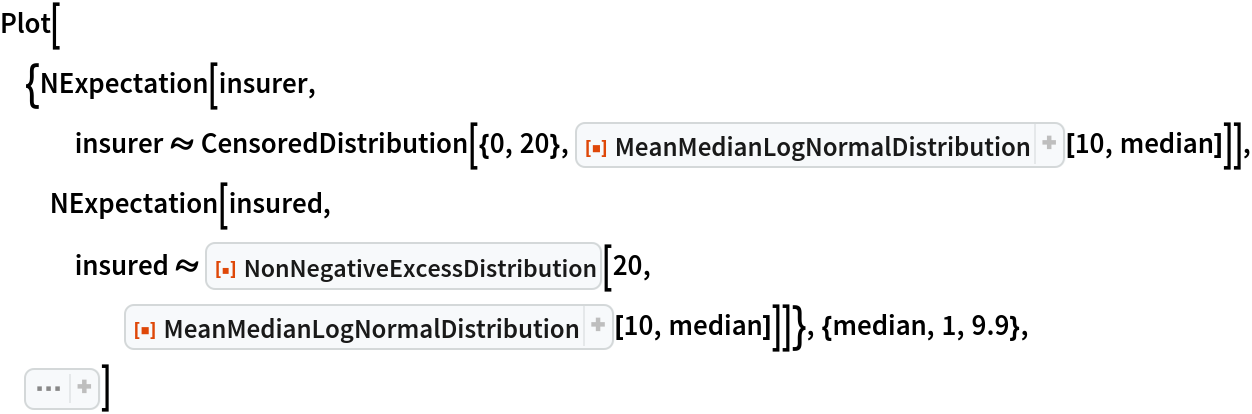Out=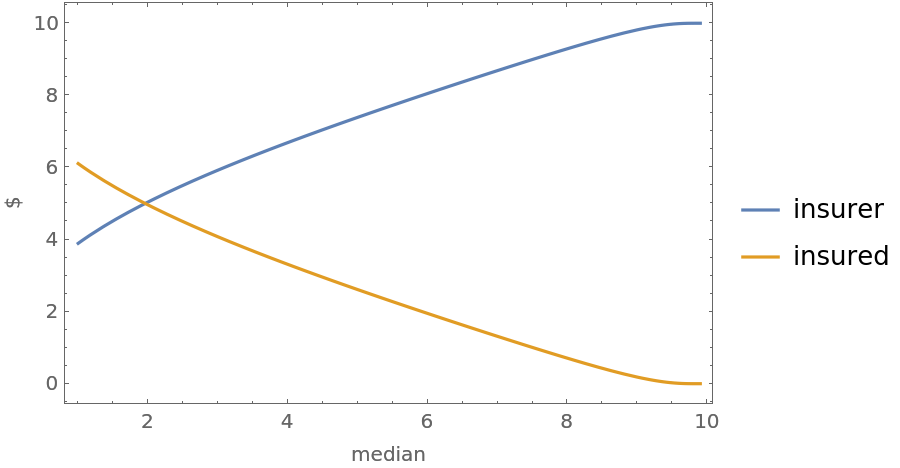Consider how risk aversion alters the analysis. Suppose that when the insured considers two outputs from a distribution, they focus on the worst case:

 In:=Out=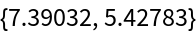In:=Out=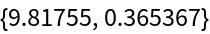Seth J. Chandler

## Version History

• 1.0.0 – 08 February 2023